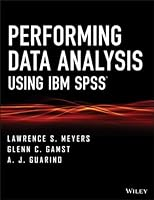# Performing Data Analysis Using IBM SPSS## Book Description

Features easy-to-follow insight and clear guidelines to perform data analysis using SPSS®

Performing Data Analysis Using IBM SPSS® uniquely addresses the presented statistical procedures with an example problem, detailed analysis, and the related data sets. Data entry procedures, variable naming, and step-by-step instructions for all analyses are provided in addition to IBM SPSS point-and-click methods, including details on how to view and manipulate output.

Designed as a user’s guide for students and other interested readers to perform statistical data analysis with IBM SPSS, this book addresses the needs, level of sophistication, and interest in introductory statistical methodology on the part of readers in social and behavioral science, business, health-related, and programs. Each chapter of Performing Data Analysis Using IBM SPSS covers a particular statistical procedure and offers the following: an example problem or analysis goal, together with a data set; IBM SPSS analysis with step-by-step analysis setup and accompanying screen shots; and IBM SPSS output with screen shots and narrative on how to read or interpret the results of the analysis.

The book provides in-depth chapter coverage of:

• IBM SPSS statistical output
• Descriptive procedures
• Score assumption evaluations
• Bivariate correlation
• Regressing (predicting) quantitative and categorical variables
• Survival analysis
• t Test
• ANOVA and ANCOVA
• Multivariate group differences
• Multidimensional scaling
• Cluster analysis
• Nonparametric procedures for frequency data

Performing Data Analysis Using IBM SPSS is an excellent text for upper-undergraduate and graduate-level students in courses on social, behavioral, and health sciences as well as secondary education, research , and statistics. Also an excellent reference, the book is ideal for professionals and researchers in the social, behavioral, and health sciences; applied statisticians; and practitioners working in industry.

Part 1. Getting Started With Ibm Spss®
Chapter 1. Introduction To Ibm Spss®
Chapter 2. Entering Data In Ibm Spss
Chapter 3. Importing Data From Excel To Ibm Spss®

Part 2. Obtaining, Editing, And Saving Statistical Output
Chapter 4. Performing Statistical Procedures In Ibm Spss®
Chapter 5. Editing Output
Chapter 6. Saving And Copying Output

Part 3. Manipulating Data
Chapter 7. Sorting And Selecting Cases
Chapter 8. Splitting Data Files
Chapter 9. Merging Data From Separate Files

Part 4. Descriptive Statistics Procedures
Chapter 10. Frequencies
Chapter 11. Descriptives
Chapter 12. Explore

Part 5. Simple Data Transformations
Chapter 13. Standardizing Variables To Z Scores
Chapter 14. Recoding Variables
Chapter 15. Visual Binning
Chapter 16. Computing New Variables
Chapter 17. Transforming Dates To Age

Part 6. Evaluating Score Distribution Assumptions
Chapter 18. Detecting Univariate Outliers
Chapter 19. Detecting Multivariate Outliers
Chapter 20. Assessing Distribution Shape: Normality, Skewness, And Kurtosis
Chapter 21. Transforming Data To Remedy Statistical Assumption Violations

Part 7. Bivariate Correlation
Chapter 22. Pearson Correlation
Chapter 23. Spearman Rho And Kendall Tau-B Rank-Order Correlations

Part 8. Regressing (Predicting) Quantitative Variables
Chapter 24. Simple Linear Regression
Chapter 25. Centering The Predictor Variable In Simple Linear Regression
Chapter 26. Multiple Linear Regression
Chapter 27. Hierarchical Linear Regression
Chapter 28. Polynomial Regression
Chapter 29. Multilevel Modeling

Part 9. Regressing (Predicting) Categorical Variables
Chapter 30. Binary Logistic Regression
Chapter 31. Roc Analysis
Chapter 32. Multinominal Logistic Regression

Part 10. Survival Analysis
Chapter 33. Survival Analysis: Life Tables
Chapter 34. The Kaplan–Meier Survival Analysis
Chapter 35. Cox Regression

Part 11. Reliability As A Gauge Of Measurement Quality
Chapter 36. Reliability Analysis: Internal Consistency
Chapter 37. Reliability Analysis: Assessing Rater Consistency

Part 12. Analysis Of Structure
Chapter 38. Principal Components And Factor Analysis
Chapter 39. Confirmatory Factor Analysis

Part 13. Evaluating Causal (Predictive) Models
Chapter 40. Simple Mediation
Chapter 41. Path Analysis Using Multiple Regression
Chapter 42. Path Analysis Using Structural Equation Modeling
Chapter 43. Structural Equation Modeling

Part 14. T Test
Chapter 44. One-Sample T Test
Chapter 45. Independent-Samples T Test
Chapter 46. Paired-Samples T Test

Part 15. Univariate Group Differences: Anova And Ancova
Chapter 47. One-Way Between-Subjects Anova
Chapter 48. Polynomial Trend Analysis
Chapter 49. One-Way Between-Subjects Ancova
Chapter 50. Two-Way Between-Subjects Anova
Chapter 51. One-Way Within-Subjects Anova
Chapter 52. Repeated Measures Using Linear Mixed Models
Chapter 53. Two-Way Mixed Anova

Part 16. Multivariate Group Differences: Manova And Discriminant Function Analysis
Chapter 54. One-Way Between-Subjects Manova
Chapter 55. Discriminant Function Analysis
Chapter 56. Two-Way Between-Subjects Manova

Part 17. Multidimensional Scaling
Chapter 57. Multidimensional Scaling: Classical Metric
Chapter 58. Multidimensional Scaling: Metric Weighted

Part 18. Cluster Analysis
Chapter 59. Hierarchical Cluster Analysis
Chapter 60. K-Means Cluster Analysis

Part 19. Nonparametric Procedures For Analyzing Frequency Data
Chapter 61. Single-Sample Binomial And Chi-Square Tests: Binary Categories
Chapter 62. Single-Sample (One-Way) Multinominal Chi-Square Tests
Chapter 63. Two-Way Chi-Square Test Of Independence
Chapter 64. Analysis
Chapter 65. Chi-Square Layers
Chapter 66. Hierarchical Loglinear Analysis